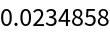#Function Repository Resource:

# MortalityConvert

Convert vector descriptions of mortality into another representation

Contributed by: Seth J. Chandler
 ResourceFunction["MortalityConvert"][vector,from→to] takes a vector representing mortality using the form represented by from and converts it to the form represented by to.

## Details and Options

from and to must be different strings taken from the set {"Hazard","Survival","Probability","CDF"}. Failure to do so generates an error message and returns \$Failed.
The numeric values of a survival representation of mortality should be monotonically decreasing and the numeric values of a CDF representation should be monotonically increasing. Failure to satisfy these requirements generates an Undefined result.
Any numeric values for the hazard representation of mortality should be between 0 and 1 inclusive. Failure to satisfy this requirement generates an Undefined result.
Any numeric values for the probability representation of mortality should be between 0 and 1 and should sum to 1. Failure to satisfy either of these requirements generates an Undefined result.
ResourceFunction["MortalityConvert"] only works on discrete distributions and it assumes the time intervals between elements of the supplied vector are the same.

## Examples

### Basic Examples

Convert a description of mortality using "Hazard" into an equivalent description using "Survival":

 In:=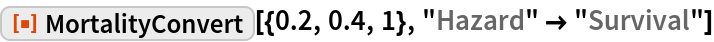Out=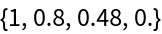Convert the previous description of mortality using "Survival" into an equivalent description using "Probability":

 In:=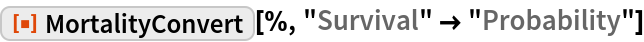Out=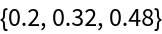Convert the previous description of mortality using "Probability" into an equivalent description using a cumulative distribution function (CDF):

 In:=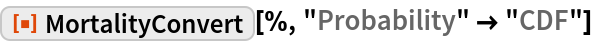Out=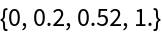Convert the previous description of mortality using a cumulative distribution function into an equivalent description using "Hazard" (returning, thereby, to the original input):

 In:=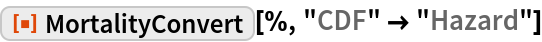Out=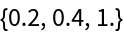### Scope

If a symbolic vector is used as input, a conditional expression is generated:

 In:=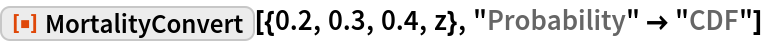Out=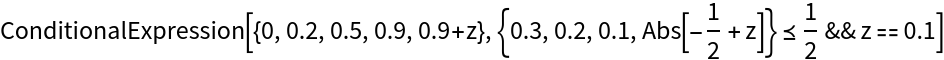The vector may be purely symbolic:

 In:=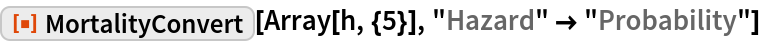Out=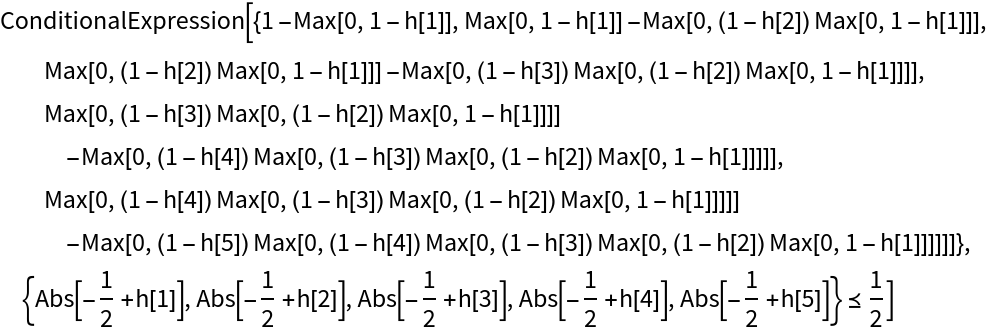Conversions from one description to the same description return the original data:

 In:=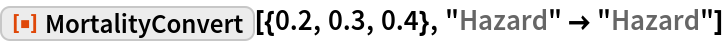Out=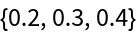If an impossible vector is used as input, the result is Undefined:

 In:=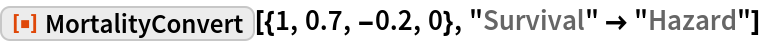Out=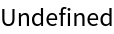Unlisted representations (e.g. from spelling errors) generate a \$Failed and an explanatory error message:

 In:=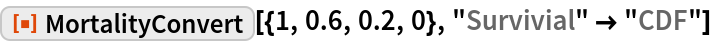Out=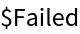### Applications

Compute the expected value of death benefits with a mortality table given as a survival vector and a 100% interest rate, which is equivalent to a 50% discount rate:

 In:=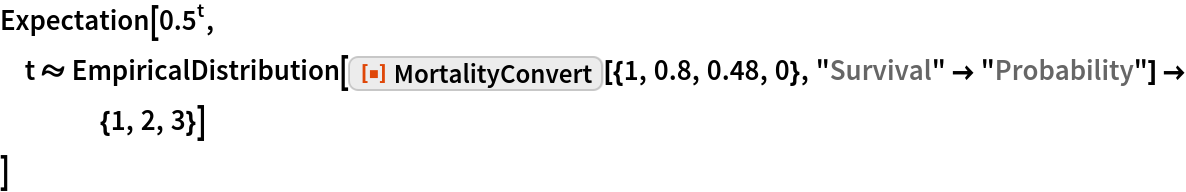Out=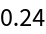Do the same computation using TimeValue and interest rates:

 In:=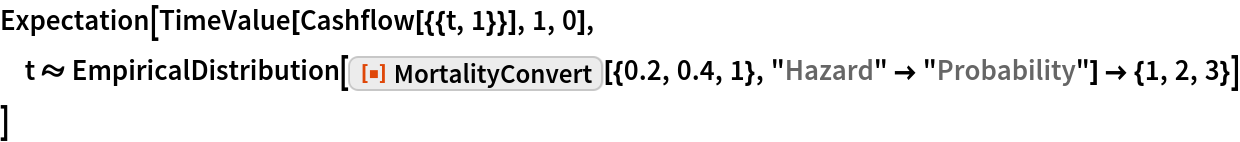Out=Compute the expected value of a premium of \$1 paid at the beginning of each year the person is alive with a mortality table given as a hazard vector and a 100% interest rate:

 In:=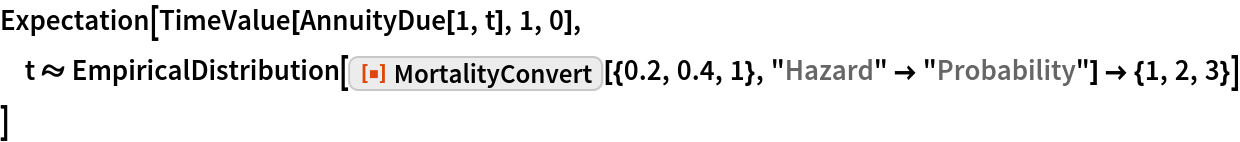Out=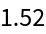Compute the premium required to set the expected value of premiums equal to the expected value of death benefits given mortality stated in different ways:

 In:=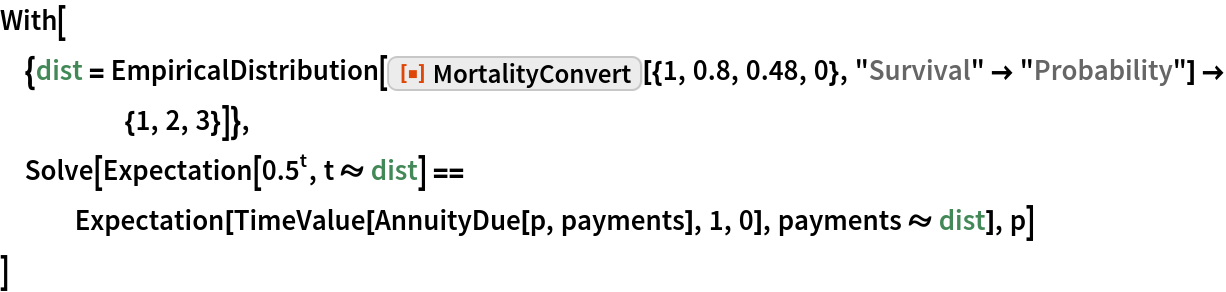Out=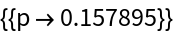### Neat Examples

Compute the prospective reserve on an insurance policy at time z for which the insurer receives the same premium each year so long as the insured is alive, such that at the outset of the policy the expected time value of the premiums is equal to the expected time value of the death benefits:

 In:=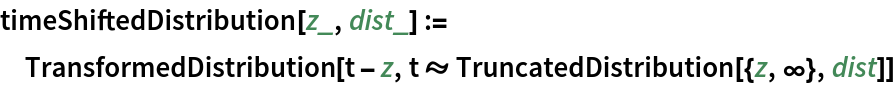In:=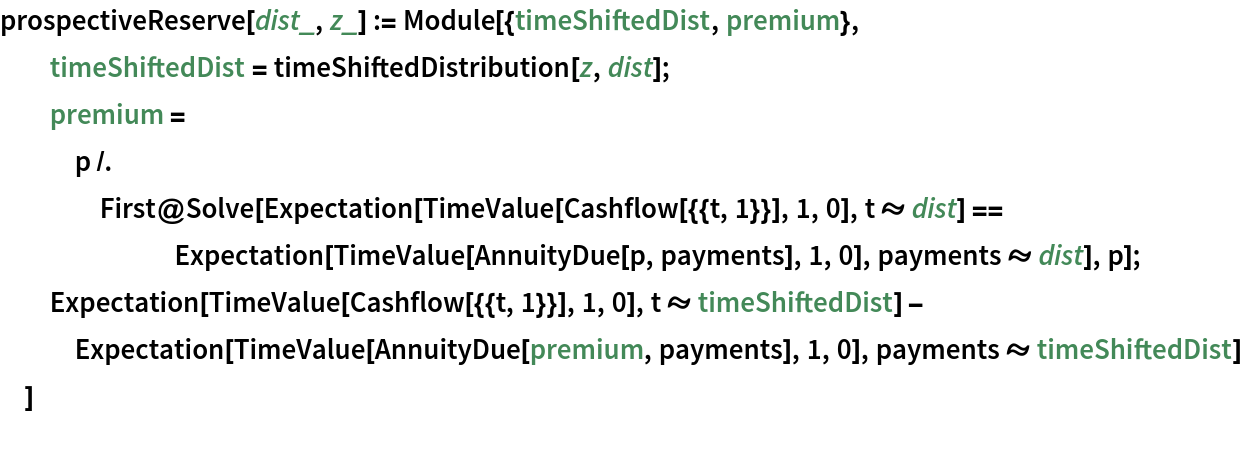In:=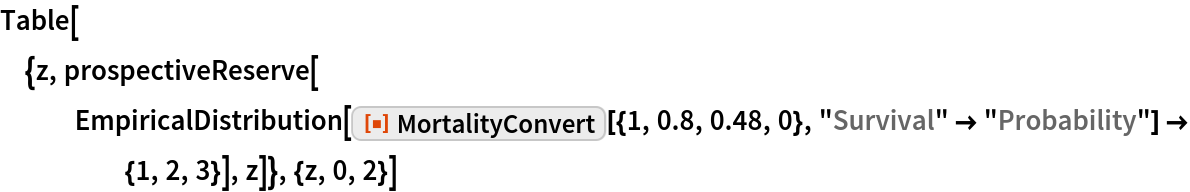Out=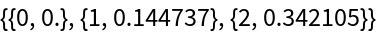An insurer pays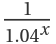if risk 1 first materializes at time x and pays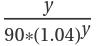if risk 2 first materializes at time y, where the materializations of the risks are independent and the distribution of time at which either materializes is given as a list of hazard probabilities. Compute the expected present value of the insurer’s payout, assuming a 5% interest rate:

 In:=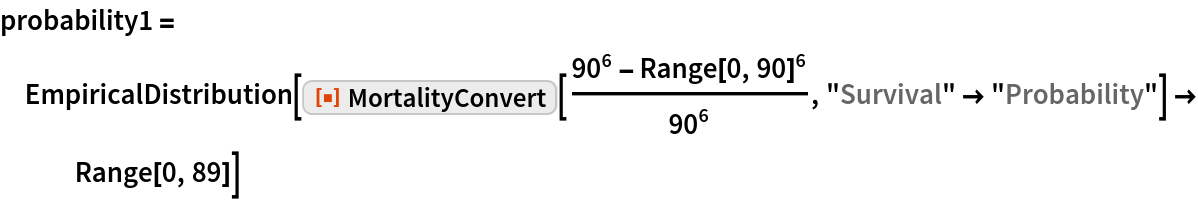Out=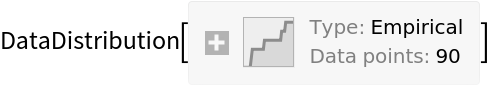In:=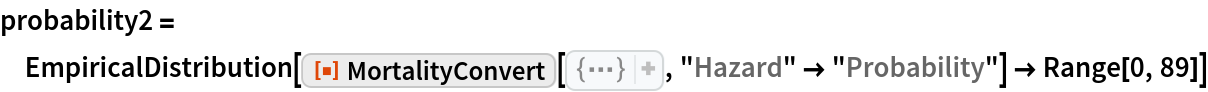Out=In:=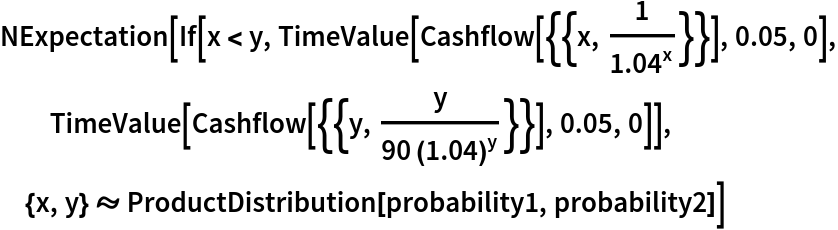Out=